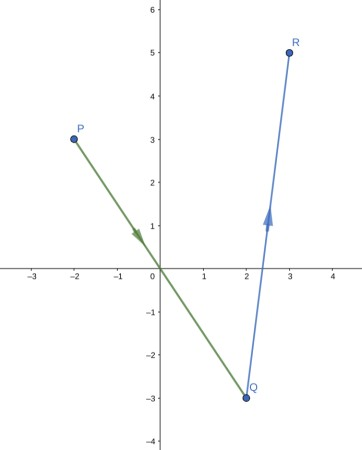# Give a set of parametric equations that describes the following curves. Graph the curve and...

## Question:

Give a set of parametric equations that describes the following curves. Graph the curve and indicate the positive orientation.

The piecewise linear path from P(-2, 3) to Q(2, -3) to R(3, 5), using parameter values {eq}0 \leq t \leq 2 {/eq}.

## Parametric Curves of Line Segments:

To find a parametrization of a line segment between two given points {eq}\displaystyle (x_0, y_0, z_0), (x_1, y_1, z_1) {/eq}

we will determine the direction vector of the line passing through the two points,

and write a parametrization as {eq}\displaystyle \mathbf{r}(t)=\mathbf{t_0}+t\mathbf{r}'(t), a\leq t\leq b. {/eq}

If the domain of the parameter t is changed, then we will take multiples of the tangent vector components,

{eq}\displaystyle \mathbf{r}'(t)=\langle a (x_1-x_0), b(y_1-y_0), c(z_1-z_0)\rangle, {/eq} in order to satisfy the starting and ending points of the line segment.

To find a parametric description of the line segments {eq}\displaystyle P(-2, 3) \text{ to } Q(2, -3)\text{ to }R(3, 5), {/eq} using the parameter {eq}\displaystyle 0\leq t\leq 2, {/eq}

we will obtain the direction vector of each line segment, first.

The direction vector for PQ is {eq}\displaystyle \vec{PQ}=\langle 4, -6\rangle. {/eq}

Next, to obtain a parametrization such that at {eq}\displaystyle t=0, {/eq} the curve to start at P and for {eq}\displaystyle t=2, {/eq} the curve to end at Q,

we will use the following parametrization, {eq}\displaystyle \mathbf{r}_1(t) =\langle -2+4at, 3-6bt\rangle, {/eq}

where {eq}\displaystyle a, b {/eq} are determined from the condition of the end-point {eq}\displaystyle t=2 {/eq} to be Q.

{eq}\displaystyle \begin{align}\mathbf{r}_1(2)&=\langle 2,-3\rangle \iff \begin{cases} -2+8a=2\\ 3-12b=-3 \end{cases}\iff a=\frac{1}{2}, b=\frac{1}{2}\\ &\text{ therefore, the equation is } \boxed{ \mathbf{r}_1(t)=\left\langle -2+2t, 3-3t\ \right\rangle, 0\leq t\leq 2}. \end{align} {/eq}

For a parametric description of the line segment from {eq}\displaystyle Q(2, -3) \text{ to } R(3, 5), {/eq} using the parameter {eq}\displaystyle 0\leq t\leq 2, {/eq}

we will obtain direction vector for QR, which is {eq}\displaystyle \vec{QR}=\langle 1, 8\rangle. {/eq}

Next, to obtain a parametrization such that at {eq}\displaystyle t=0, {/eq} the curve to start at Q and for {eq}\displaystyle t=2, {/eq} the curve to end at R,

we will use the following parametrization, {eq}\displaystyle \mathbf{r}_2(t) =\langle 2+at, -3+8bt\rangle, {/eq}

where {eq}\displaystyle a, b {/eq} are determined from the condition of the end-point {eq}\displaystyle t=2 {/eq} to be R.

{eq}\displaystyle \begin{align}\mathbf{r}_2(2)&=\langle 3,5\rangle \iff \begin{cases} 2+2a=3\\ -3+16b=5 \end{cases}\iff a=\frac{1}{2}, b=\frac{1}{2}\\ &\text{ therefore, the equation is } \boxed{ \mathbf{r}_2(t)=\left\langle 2+\frac{t}{2}, -3+4t\ \right\rangle, 0\leq t\leq 2}. \end{align} {/eq}

The parametric curves obtained above are used to obtain the plot below, where the directions on the two line segments are shown with arrows.